# Civil Engineering - Advanced Surveying

### Exercise :: Advanced Surveying - Section 1

41.

The angle between the direction of star and the direction of earth's axis of rotation is called

 A. co-declination B. co-latitude C. declination D. latitude.

Explanation:

No answer description available for this question. Let us discuss.

42.

If v, t and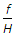are the ground speed of the aircraft, the shutter speed of the camera and the scale of the photograph respectively, then the amount of image displacement

 A.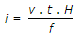B.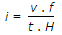C.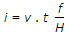D.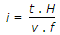Explanation:

No answer description available for this question. Let us discuss.

43.

Pick up the correct statement from the following :

 A. The angle between the plane of the negative and the horizontal plane containing perspective axis is the tilt of the photograph B. The direction of maximum tilt is defined by the photo principal line C. The principal plane is truly vertical plane which contains perspective centre as well as principal point and plumb point D. All the above.

Explanation:

No answer description available for this question. Let us discuss.

44.

The circle in which a plane tangent to the earth's surface at the point of observation, intersects the celestial sphere, is called

 A. visible horizon B. sensible horizon C. celestial horizon D. true horizon E. none of the above.

Explanation:

No answer description available for this question. Let us discuss.

45.

If θ and δ be the latitude of an observer and declination of a heavenly body respectively, the upper culmination of the body will be south of zenith if its zenith distance, is

 A. δ - θ B. θ - δ C. θ + δ D.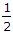(θ - δ).